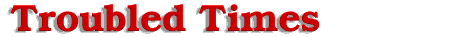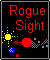#Transit Time

Transit time to Earth passage from the Sun is now the key element to find. This new factor to be solved will determine the base date of the table for Sun passage of the 12th planet. To find the position of Earth along its orbital track, I will use another given factor. The 12th planet passes to the left of the Sun about half way between the orbits of Mercury and Venus or approximately 50 million miles. Using trigonometry, we will set up a right triangle at the location where the 12th planet passes the Sun. A line is projected from the 12th planet's location to an intercept point on the Earth's orbital path or the projected passage point, its length unknown. This line traces the 12th planet's orbital path. We will designate this, the first leg of the right triangle formed at the location of the 12th planet. A second line is projected perpendicular to the first leg starting from the same location of the 12th planet to the Sun and ends there. This second leg of the right triangle from the 12th planet to the Sun, is 50 million miles. The hypotenuse leg of the right triangle, connecting the Sun and the Earth orbital intercept point is 92.95 million miles in length. It is the standard distance from the Sun to the Earth. Using the inverse cosine function, on the result of the adjacent leg over the hypotenuse the angle, we can find the angle between the 12th and the Earth at the Sun. This yields an answer equivalent to 57.45 degrees.

The equation proceeds as follows:

cosine -1 [adjacent leg / hypotenuse] = angle
cosine -1 [ 50/92.950] = angle
cosine -1 [ .5379236] = angle
57.45 degrees = angle

Lets solve for the opposite leg or the first leg of the right triangle for angle between the 12th planet and the Earth intercept point at location of the Sun. The distance between the 12th planet and the Earth orbit intercept point using the sine function of the angle equals the opposite leg over the hypotenuse, yielding 78.349 million miles.

The equation proceeds as follows:

opposite leg / hypotenuse = sine[angle]
opposite leg = sine[angle] * hypotenuse
opposite leg = sine[57.45] * 92.95
opposite leg = .84292 * 92.95
opposite leg or travel distance
to Earth for the 12th planet is 78.349 million miles.

All the necessary variables have now been found to solve for Time (t), the time it takes the 12th planet to move between Sun passage and Earth passage. Solving for Time (t), the time it takes the 12th planet to move between Sun passage and Earth passage, Distance(final) is equal to {3.56 units - 0.0213333 S-P units [78.4 million miles]) or 3.5386667 units, Distance(initial) is equal to 3.56 S-P units, and k equals -0.005761. The equation yields a time (t) of 1.0433 weeks after passing the Sun.

The equation proceeds as follows:

Distance(final) = Distance(initial) * e ^ [(k) * (t)]
LN [Distance(final) / Distance(initial)] = (k) * (t)
LN [Distance(final) / Distance(initial)] / k = t
LN [3.5386667 / 3.56] / -0.005761 = t
LN [0.9940075] / -0.005761 = t
-0.006010527 / -0.005761 = t
1.0433 weeks = t
the time interval from Sun passage to Earth passage.

So if the 12th planet Earth passage date is May 15, 2003. Then the base date or Sun passage would be May 7, 2003 or 1.0433 weeks prior for the distance table. You can adjust your dates accordingly if a more accurate Earth passage date is predicted.

In conclusion two significant issues should become clearer in your mind. First, visibility of the 12th planet will come too little and too late. Second, the storms prior to Earth passage are going to be far worse and more powerful than the present picture painted in our minds. One only has to look at the weather changes already initiated from an out of phase magnetic alignment produced by movement of the 12th planet on only 3 tenths of 1% from the mid point to our Sun by January of the year 2000. All primary data and some concepts used in the previous text can be referenced in the science section of Zeta Talk under Comet Orbit, Entry Angle and Repulsion Force. The chart illustrating the orbital passage of the 12th planet through the solar system is located in this TOPIC on the Sagittarius page. I would like to give thanks for the guidance given in my life from up above. I will always appreciate the warmth and light of the Sun. The breeze that blows on my face. What we take so easily for granted, some worlds may only experience only a few precious moments of it.

Offered by Robert.